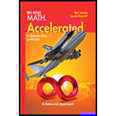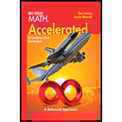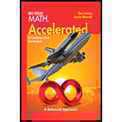page contents

Welcome To Our Study of Math!

 7 - 1 Accelerated Math   (Chapters 1 through 16)   Integers and Rational Numbers Equations and Inequalities Ratios and Proportions Percents Geometry Probability Real Numbers The Pythagorean Theorem Exponents and Scientific Notation7 - 2 Math    (Chapters 1 through 10)   Integers and Rational Numbers Equations and Inequalities Ratios and Proportions Percents Geometry Probability7 - 3 Math   (Chapters 1 through 10)   Integers and Rational Numbers  Equations and Inequalities Ratios and Proportions Percents Geometry ProbabilityAll Test and Quiz Reviews, as well as Assignments, will be Posted on Student's Google Classroom Page.